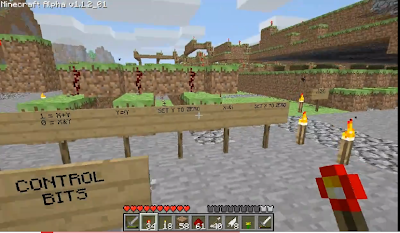## Wednesday, September 29, 2010

### Are you listening, pig?I'd just like to share some recommendations that are all connected by the theme of visual and concrete computing. In the last few years I've become more and more convinced that (in the United States at least) our educational system is almost completely failing to provide adequate skills in computing - both the theory and practice of computer science. This is sad because I think there are many fun and interesting ways to learn about programming, logic, and algorithms. Here are some examples:

1. LOGO. When I was in elementary school I was exposed to Logo, a very visual platform for learning programming. If you have a child, make sure you check out an implementation and show your kid. No matter what computer you have, its almost certain that there are several free Logo programs for it.

2. Similar in spirit to Logo, but maybe more fun and less deep is the game Light-Bot at Armor Games. You can drag and drop visual program elements to write a program that instructs a robot to complete a variety of puzzles. Very fun, well done.

3. There are novels that glamorize hackers and computing to some extent, but nothing I've seen compares to the plot in Souls in the Great Machine by Sean McMullen. The plot is brilliant and complicated, but all you need to know is that they enslave all the mathematicians to create a giant human computer.

4. I was reminded of Eyes of the Calculor by this fantastic video on youtube. The creator has implemented a gigantic arithmetic unit within the virtual Minecraft environment. He mentions he was inspired by the book The Elements of Computing Systems: Building a Modern Computer from First Principles. I hadn't heard of that before but I will definitely take a look. If you watch the video you'll get the title of this post.

## Sunday, September 12, 2010

### Torus knots in Sage with Tachyon and @parallelAfter working a bit more on a patch improving the Tachyon raytracer in Sage, I made a movie of a torus knot. The code below was parallelized to use 20 cores at a time on one of the Sage Foundation's lovely Sun Fire X4450s. Frames were animated with ffmpeg. Blogger might degrade the video, so I recommend downloading the original here.

pk = 3qk = 23res = 180trad = .5lab = 'tk_' + str(pk) + '_' + str(qk)def tknot(theta):    return [(2 + trad*cos(qk*theta/pk))*cos(theta), \\(2 + trad*cos(qk*theta/pk))*sin(theta), trad*sin(qk*theta/pk)]@parallel(ncpus = 20)def tknotter(anum):    th = anum*2.0*pi/res    T = Tachyon(xres = 800, yres = 600, camera_center = (4,4,2.5), \\raydepth=12, antialiasing=2)    loc = tknot(th)    nloc = [abs(x)/norm(vector(loc)) for x in loc]    T.texture('s1',color=nloc,specular=1)    T.texture('p1',color=(.5,.5,.5))    T.sphere(loc,.05,'s1')    ci = 0    for sth in srange(0,th,2*pi/res):        e1 = tknot(sth)        e2 = tknot(sth+2*pi/res)        ts = [abs(x)/norm(vector(e1)) for x in e1]        T.texture('t'+str(ci), color=ts)        T.fcylinder(e1, e2, .025,'t'+str(ci))        ci += 1    T.plane((0,0,-4),(0,0,1),'p1')    T.light((6,6,11),.1,(1,1,1))    T.light((5.9,5.9,11),.1,(1,1,1))    T.save(DATA+lab+'%03d.png'%anum)    return 1qin = range(pk*res)s = list(tknotter(qin))for i in range(40):    r2 = os.system('cp '+DATA+lab+'%03d.png '%(pk*res-1) + DATA+lab+'%03d.png'%(pk*res+i))anum = qin[-1] + 41th = anum*2*pi/resT = Tachyon(xres = 800, yres = 600, camera_center = (5,5,3))T.save(DATA+lab+'%03d.png'%anum)

The animation was then done with the following code; if you want to do something similar you would have to use the appropriate path to your ffmpeg executable.
import subprocesssubprocess.call('/home/mhampton/ffmpeg/ffmpeg -qmax 2 -i ' + \\DATA + 'v%3d.png ./t2vest.mp4', shell=True, stdout=file('/dev/null','w'),stderr=file('/dev/null','w'))

### Flight in Curved SpacesI was recently reminiscing about the movie Not Knot, and began thinking about recreating some of the hyperbolic scenes. But then today I stumbled upon a really nice application that does exactly what I was thinking about and more:

http://www.geometrygames.org/CurvedSpaces/index.htmlT = Tachyon(xres=800,antialiasing=24, raydepth=12, projection = 'perspective_dof', focallength = '1.0', aperture = '.005')T.light((0,5,7),1.0,(1,1,1))T.texture('t1', opacity=1, specular = .3)T.texture('t2', opacity=1, specular = .3, color = (0,0,1))T.texture('t3', opacity = 1, specular = 1, color = (1,.8,1), diffuse=0.2)T.plane((0,0,-1),(0,0,1),'t3')ttlist = ['t1','t2']tt = 't1'T.cylinder((0,0,.1),(1,1/3,0),.05,'t3')for q in srange(-3,100,.15):    if tt == 't1':        tt = 't2'    else:        tt = 't1'    T.sphere((q,q/3+.3*sin(3*q),.1+.3*cos(3*q)), .1, tt)T.show()